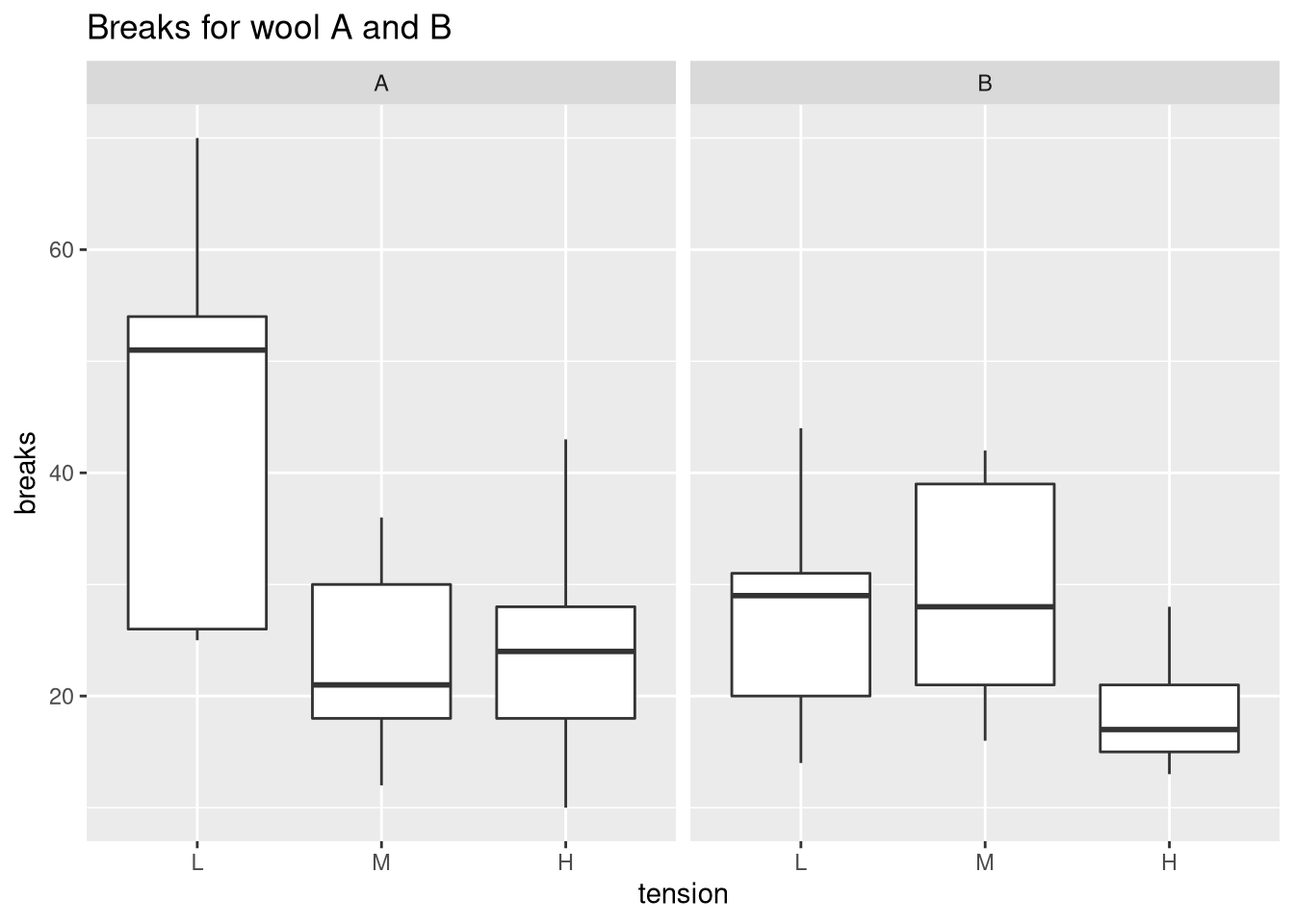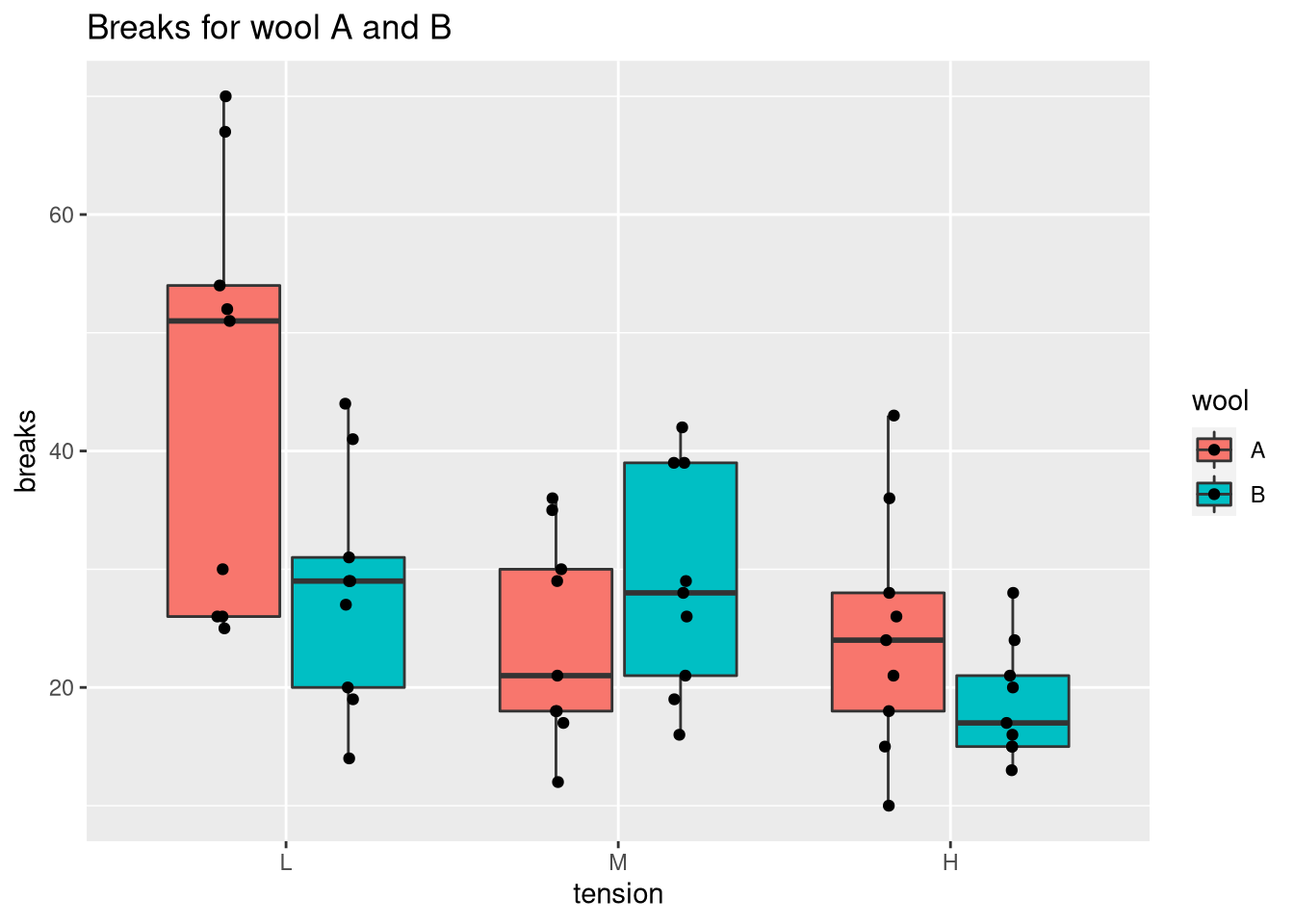# Comparing Medians and Inter-Quartile Ranges Using the Box Plot

(This article was first published on R on datascienceblog.net: R for Data Science, and kindly contributed to R-bloggers)

The box plot is useful for comparing the quartiles of quantitative variables. More specifically, lower and upper ends of a box (the hinges) are defined by the first (Q1) and third quartile (Q3). The median (Q2) is shown as a horizontal line within the box. Additionally, outliers are indicated by the whiskers of the boxes whose definition is implementation-dependent. For example, in `geom_boxplot` of ggplot2, whiskers are defined by the inter-quartile range (IQR = Q3 – Q1), extending no further than 1.5 * IQR.

## Creating a box plot in native R

We will use the warpbreaks data set to exemplify the use of box plots. In native R, a box plot can be obtained via `boxplot`.

``````data(warpbreaks)
# create positions for tick marks, one more than number of bars
x <- warpbreaks\$breaks
# create labels
x.labels <- paste0(warpbreaks\$wool, "-", warpbreaks\$tension)
# specify colors for groups
group.cols <- c("darkred", "red", "darksalmon",
"darkblue", "blue", "lightblue")
cols <- c(rep(group.cols, 9), rep(group.cols, 9),
rep(group.cols, 9), rep(group.cols, 9),
rep(group.cols, 9), rep(group.cols, 9))
boxplot(x ~ warpbreaks\$wool + warpbreaks\$tension, col = group.cols)
legend("topright", legend = c(unique(x.labels)),
col = group.cols, pch = 20)``````## Creating a box plot with ggplot

We could compare the tensions for each type of wool using `facet_wrap` in the following way:

``````library(ggplot2)
ggplot(warpbreaks, aes(x = tension, y = breaks)) +
geom_boxplot() + facet_wrap(.~wool) +
ggtitle("Breaks for wool A and B")````````````ggplot(warpbreaks, aes(x = tension, y = breaks, fill = wool)) +
geom_boxplot() +
ggtitle("Breaks for wool A and B")``````### Showing all points

To view the individual measurements associated with the box plot, we set `outlier.shape = NA` to prevent duplicates and call `geom_point`.

``````ggplot(warpbreaks, aes(x = tension, y = breaks, fill = wool)) +
geom_boxplot(outlier.shape = NA) +
ggtitle("Breaks for wool A and B") +
# dodge points horizontally (there are two bars per tick)
# and jitter points horizontally so that they don't overlap
geom_point(position = position_jitterdodge(jitter.width = 0.1))``````Showing all the points helps us to identify whether the sample size is sufficient. In this case, most pairs of wool and tension exhibit high variabilities (especially wool A with tension L). Thus, the question would be whether this level of variability is inherent to the data or a result of the small number of samples (n = 9). Note that you can combine a box plot with a beeswarm plot to optimize the locations of the points.

To leave a comment for the author, please follow the link and comment on their blog: R on datascienceblog.net: R for Data Science.

R-bloggers.com offers daily e-mail updates about R news and tutorials on topics such as: Data science, Big Data, R jobs, visualization (ggplot2, Boxplots, maps, animation), programming (RStudio, Sweave, LaTeX, SQL, Eclipse, git, hadoop, Web Scraping) statistics (regression, PCA, time series, trading) and more...

If you got this far, why not subscribe for updates from the site? Choose your flavor: e-mail, twitter, RSS, or facebook...